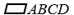Chapter 4.1, Problem 10E### Elementary Geometry for College St...

6th Edition
Daniel C. Alexander + 1 other
ISBN: 9781285195698

#### Solutions

Chapter
Section### Elementary Geometry for College St...

6th Edition
Daniel C. Alexander + 1 other
ISBN: 9781285195698
Textbook Problem
20 views

# Given that m ∠ A = 2 x + 3 , and m ∠ C = 3 x - 27 , find the measure of each angle of.To determine

To find:

The measure of each angle of ABCD.

Explanation

Given:

Given that mA=2x+3, and mC=3x-27, and the ABCD as shown below.

Corollary:

1. The opposite angles of a parallelogram are congruent.

2. Two consecutive angles of a parallelogram are supplementary.

In the given parallelogram ABCD, given that mA=2x+3, and mC=3x-27.

As per the corollary that the opposite angles of a parallelogram are congruent, the opposite angles mA and mC are equal or mA=mC and also mB and mD are equal or mB=mD.

To find the value of x, substitute mA=2x+3, and mC=3x-27 in mA=mC

2x+3=3x-27

2x-3x=-27-3

-x=-30

x=30

To find mA, substitute x =30 in mA=

### Still sussing out bartleby?

Check out a sample textbook solution.

See a sample solution

#### The Solution to Your Study Problems

Bartleby provides explanations to thousands of textbook problems written by our experts, many with advanced degrees!

Get Started

#### An integral for the solid obtained by rotating the region at the right about the y-axis is:

Study Guide for Stewart's Single Variable Calculus: Early Transcendentals, 8th

#### True or False: Du f(a, b) = ∇(a, b) · u.

Study Guide for Stewart's Multivariable Calculus, 8th

#### Explain the role of voluntary participation in informed consent.

Research Methods for the Behavioral Sciences (MindTap Course List)